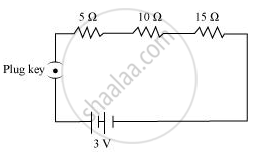# Draw a Schematic Diagram of an Electric Circuit Consisting of a Battery of Two Cells Each of 1.5 V, 5 ω, 10 ω and 15 ω Resistors and a Plug Key, All Connected in Series. - Science

#### Question

Draw a schematic diagram of an electric circuit consisting of a battery of two cells each of 1.5 V, 5 Ω, 10 Ω and 15 Ω resistors and a plug key, all connected in series.

#### Solution

The emf of each cell is 1.5 V.

When the two cells are connected in series, the emf of the battery becomes (1.5 + 1.5) V = 3 V.

Therefore, the schematic diagram of the series circuit is as follows:Is there an error in this question or solution?

#### Video TutorialsVIEW ALL 

Draw a Schematic Diagram of an Electric Circuit Consisting of a Battery of Two Cells Each of 1.5 V, 5 ω, 10 ω and 15 ω Resistors and a Plug Key, All Connected in Series. Concept: Electric Current and Circuit.